## Example Questions

### Example Question #1 : How To Find The Perimeter Of A Rectangle

A rectangle has a width of 2x. If the length is five more than 150% of the width, what is the perimeter of the rectangle?

5x + 10

10(x + 1)

6x2 + 5

6x2 + 10x

5x + 5

10(x + 1)

Explanation:

Given that w = 2x and l = 1.5w + 5, a substitution will show that l = 1.5(2x) + 5 = 3x + 5.

P = 2w + 2l = 2(2x) + 2(3x + 5) = 4x + 6x + 10 = 10x + 10 = 10(x + 1)

### Example Question #1 : How To Find The Perimeter Of A Rectangle

Find the perimeter of a rectangle with width 7 and length 9.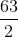Explanation:

To solve, simply use the formula for the perimeter of a rectangle.

Substitute in the width of seven and the length of nine.

Thus,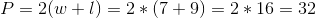### Example Question #201 : Geometry

Find the perimeter of a rectangle whose side lengths are 1 and 2.Explanation:

To solve, simply use the formula for the perimeter of a rectangle. Thus,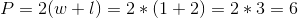### Example Question #1 : How To Find The Perimeter Of A Rectangle

Find the perimeter of a rectangle with width 6 and length 9.Explanation:

To solve, simply use the formula for the perimeter.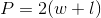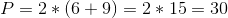Another way to solve this problem is to add up all of the sides. Remember that even though only two values are given, a rectangle has 4 sides. Thus,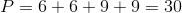### Example Question #2 : How To Find The Perimeter Of A Rectangle

You have a poster of one of your favorite bands that you are planning on putting up in your dorm room. If the poster is 3 feet tall by 1.5 feet wide, what is the perimeter of the poster?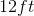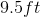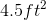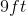Explanation:

You have a poster of one of your favorite bands that you are planning on putting up in your dorm room. If the poster is 3 feet tall by 1.5 feet wide, what is the perimeter of the poster?

Perimeter of a rectangle is found via: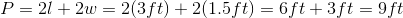### Example Question #211 : Plane Geometry

Three of the vertices of a rectangle on the coordinate plane are located at the origin,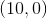, and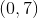. Give the perimeter of the rectangle.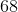Explanation:

The rectangle in question is below: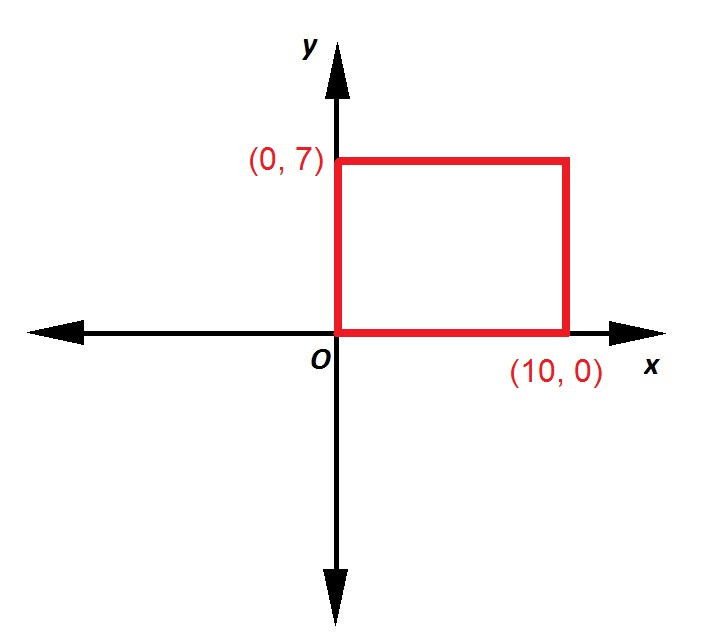The lengths of the rectangle is 10, the distance from the origin to; its width is 7, the distance from the origin to. The perimeter of a rectangle is equal to twice the sum of its length and width, so calculate: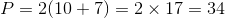.

### Example Question #1 : How To Find The Perimeter Of A Figure

A rectangular garden has an area of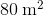. Its length ismeters longer than its width. How much fencing is needed to enclose the garden?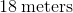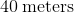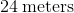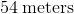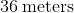Explanation:

We define the variables as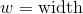and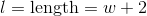.

We substitute these values into the equation for the area of a rectangle and get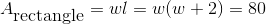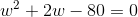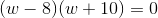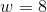or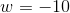Lengths cannot be negative, so the only correct answer is. If, then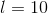Therefore,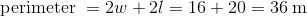.

### All SAT Math Resources